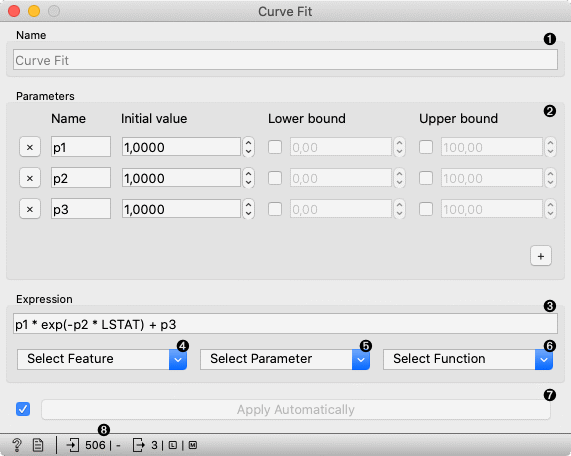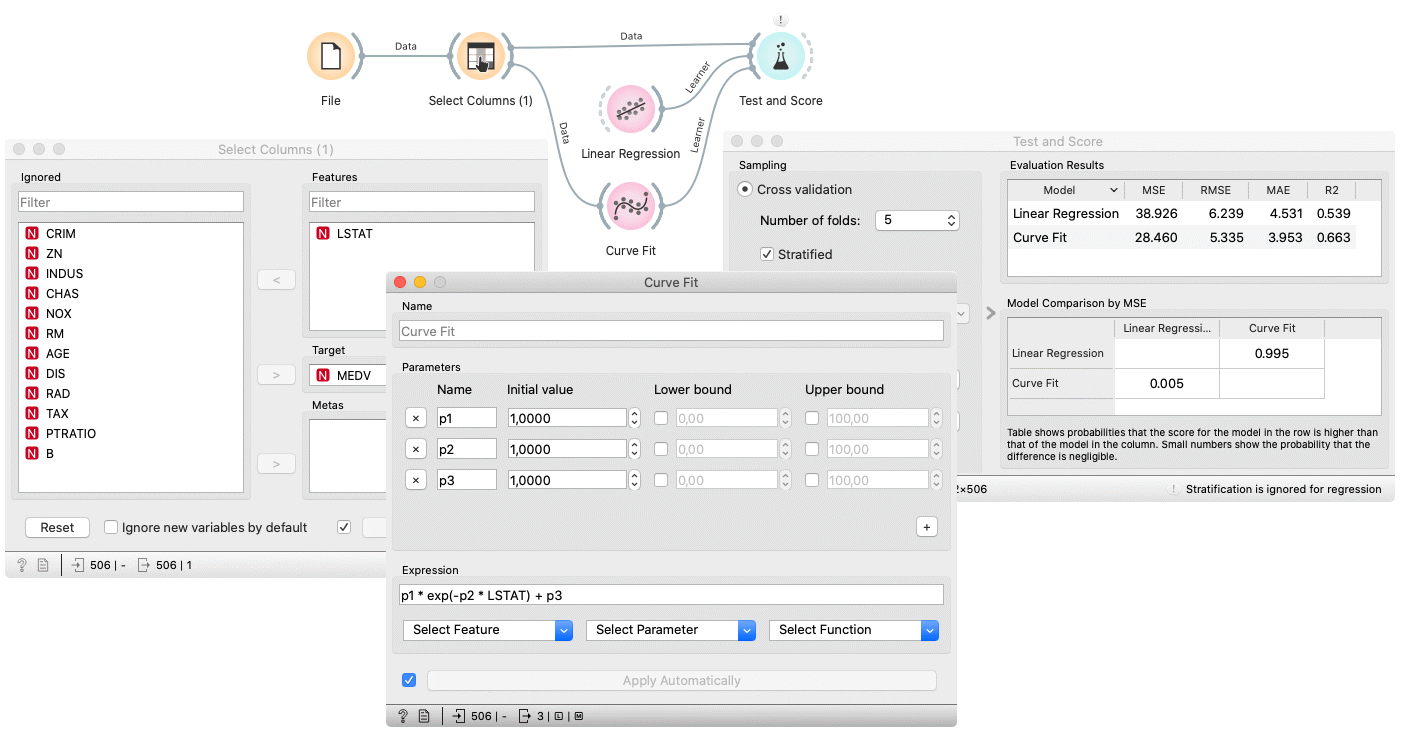# Curve Fit¶

Fit a function to data.

Inputs

• Data: input dataset

• Preprocessor: preprocessing method(s)

Outputs

• Learner: curve fit learning algorithm

• Model: trained model

• Coefficients: fitted coefficients

The Curve Fit widget fits an arbitrary function to the input data. It only works for regression tasks. The widget uses scipy.curve_fit to find the optimal values of the parameters.

The widget works only on regression tasks and only numerical features can be used for fitting.1. The learner/predictor name.

2. Introduce model parameters.

3. Input an expression in Python. The expression should consist of at least one fitting parameter.

4. Select a feature to include into the expression. Only numerical features are available.

5. Select a parameter. Only the introduced parameters are available.

6. Select a function.

7. Press Apply to commit changes. If Apply Automatically is ticked, changes are committed automatically.

8. Show help, produce a report, input/output info.

## Preprocessing¶

Curve fit uses default preprocessing when no other preprocessors are given. It executes them in the following order:

• removes instances with unknown target values

• removes empty columns

• imputes missing values with mean values

To remove default preprocessing, connect an empty Preprocess widget to the learner.

## Example¶

Below, is a simple workflow with housing dataset. Due to example simplicity we used only a single feature. Unlike the other modelling widgets, the Curve Fit needs data on the input. We trained Curve Fit and Linear Regression and evaluated their performance in Test & Score.